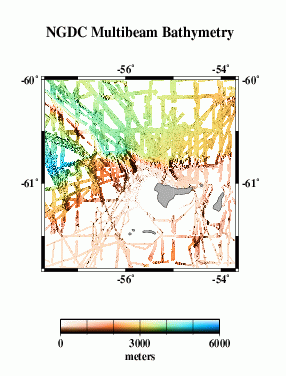# Multibeam Swath Bathymetry - Antarctic Peninsula Region

## Data### Grid Information:

• West = -57.7
• East = -53.7
• South = -61.8
• North = -60
• Grid Nodes: nx = 2001, ny = 1801
• Depth: Max = 5590 m, Min = 28 m

### GMT command line arguments:

• -R-57.7/-53.7/-61.8/-60v
• -I.002/.001

## Profile Comparisons

Here we compare profiles from swath bathymetry (A) with estimated bathymetry (B), and satellite-derived gravity anomalies (C). Because there are limited constrainted points (black dots in E) in the estimated bathymetry along the profile (red line), and because of the resolution limit of the altimeter data used in the bathymetry prediction, the estimated anomalies do not resolve short- wavelength seafloor features evident in swath bathymetry (G).

## Grid Analysis

We compare the difference between altimetry-estimated bathymetry (2 min. grid) and actual swath bathymetry (15 sec. grid) two ways, because the two are on grids of different spacing and thus may capture different length scales of bathymetric roughness.

For the lower-resolution analysis (H and I), multibeam data were averaged into corresponding 2-arc-minute blocks, and estimated bathymetry was subtracted. For the higher-resolution analysis (J and K), bilinear interpolation was used to obtain estimated bathymetry values at each point on the swath bathymetry grid, then the estimated bathymetry was subtracted.

In both cases, we find the mean difference is ~ 7 m (the swath bathymetry data are systematically deeper); the difference is about .2% of the depth. An error like this is expected because of the uncertainties in whether or not data sets used in calibration have been corrected for the variable speed of sound in seawater, errors translating uncorrected sound speeds between nominal fathoms and nominal meters, etc.

The standard deviations about the mean are 90 m for the 2 arcmin averages and 75 m for the multibeam points. This is opposite of what we expect, the standard deviations should be greater for the higher resolution differences. We do not yet know the reason for this.

Eventually, we want to do a detailed cross-spectral analysis to determine how much of the actual multibeam bathymetry is captured in the altimeter gravity field. Here, we present a very simplified model. We take the combined bathymetry (F) and simply calculate a model gravity field, assuming that all the topography has the same density and is entirely uncompensated. This model (M) does not look much like the observed gravity (L), because our assumptions are too simple, and because of the sediment cover.

The radially-averaged power spectra of the two (N) show the altimeter gravity has more power at wavelengths greater than ~30 km or so. At shorter wavelengths, noise in the altimeter data may contribute to the higher power in the altimetric gravity.